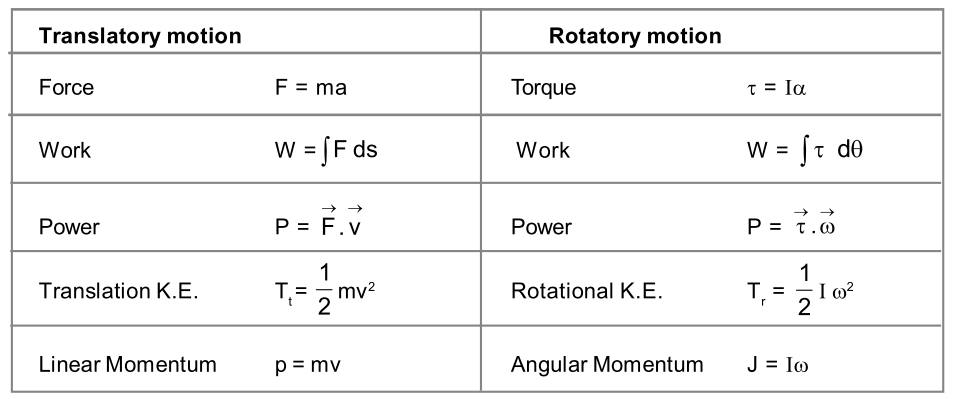Most Affordable JEE | NEET | 8,9,10 Preparation by Kota's Top IITian Doctor Faculties

# Physical Significance of Moment of Inertia for Class 11, JEE & NEETLets study about Moment of Inertia of a Rigid Body and Physical Significance of Moment of Inertia here. MOMENT OF INERTIA OF RIGID BODY It is the property of a body due to which it opposes any change in its state of rest or uniform rotation. Analytically for a particle of mass m rotating in a circle of radius R, moment of inertia of a particle of mass m about the axis of rotation is given by: $\mathrm{I}=\mathrm{mR}^{2}$ . . . . .(1) So for a body made up of number of particles (discrete distribution) of masses $m_{1}, m_{2}, \ldots \ldots \ldots$. etc. at distance $r_{1}, r_{2}, \ldots$ etc. respectively from the axis of rotation, then moment of inertia of all these particles are $m_{1} r_{1}^{2}, m_{2} r_{2}^{2}, \ldots$ and moment of intertia of body ( $I$ ) is the algebric sum of moment of inertia of all these particles- i.e. $\quad \mathrm{I}=\mathrm{m}_{1} \mathrm{r}_{1}^{2}+\mathrm{m}_{2} \mathrm{r}_{2}^{2}+\ldots \ldots+\mathrm{m}_{\mathrm{r}_{1} \mathrm{i}}^{2}+\mathrm{m}_{\mathrm{n}} \mathrm{r}_{\mathrm{n}}^{2}=\sum_{i=1}^{\mathrm{n}} \mathrm{m}_{\mathrm{r}_{1}} \mathrm{r}_{1}^{2} \ldots \ldots(2)$ $\quad \quad \mathrm{m}_{\mathrm{i}}=\mathrm{mass}$ of ith particle $r_{i}=$ distance of ith particle from axis of rotation While for a continuous distribution of mass, treating the element of mass dm as particle at position r from axis of Rotation. $$\begin{array}{ll}{\text { dI }=\mathrm{dm} \mathrm{r}^{2},} & {\text { i.e., } \quad \mathrm{I}=\int \mathrm{r}^{2} \mathrm{d} \mathrm{m}}\end{array}$$ . . . . (3) It is a scalar quantity Unit : M.K.S.: $\mathrm{kg}-\mathrm{m}^{2},$ C. G.S. : $\mathrm{gm}-\mathrm{cm}^{2}$ Dimension : $\mathrm{M}^{1} \mathrm{L}^{2} \mathrm{T}^{0}$ Moment of inertia depends on the following factors. i) Mass of body ii) Mass distribution of body or shape, size and density of body. iii) On the position of the axis of rotation. The more is the distribution of mass with respect to axis of rotation the more will be moment of inertia. Moment of Inertia does not depend on the following factors. (i) Angular velocity (ii) Angular Acceleration (iii) Torque (iv) Angular Momentum   PHYSICAL SIGNIFICANCE OF MOMENT OF INERTIA:Comparison of the expression for rotational motion with corresponding relations for translatory motion as given in table I, we get moment of inertia plays same role in rotatory motion as mass in translatory motion, i.e., if a body has large moment of inertia, it is difficult to start rotation or to stop it if rotating. Large moment of inertia also helps in keeping the motion uniform. Due to this reason stationary engines are provided with fly-wheels having large moment of inertia.   Inertia for rotational motion is moment of inertia.Introduction to Rotational Dynamics  Moment of Inertia Moment of Inertia: Perpendicular and Parallel axis theorem Radius of Gyration Law of Conservation of Angular Momentum Conservation of Angular Momentum Examples Kinetic Energy of a Rotating Body Work done in rotatory Motion Rotational Power Combine Translational and Rotational Motion Rolling without slipping Rolling on a plane surface Rolling on a Inclined Plane   For Latest updates, Subscribe our Youtube Channel

Rijul
Feb. 17, 2021, 1:24 p.m.